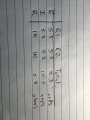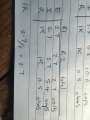# Ohms law, battery capacity, what’s the mistake in my maths

#### MissOrange

Joined Nov 7, 2014
18
Hi all and Thankyou for being such a helpful group of people.

I’m very much learning, it’s also lockdown (lack of options).

My goal is to understand how long a battery will take to drain with two leds. Circuit is a 5.3v battery, and two leds each with a 1k resistor, parallel circuit. Had them connected a couple of days now. But when I try do the maths for how long the battery should last it’s in hours not days, where am I going wrong?
5.3v battery, 2600mah each led has a voltage drop of 2.6, each resistor is 1k. So total resistance in this circuit is 500ohms (1/r1 + r2 = 0.5 and 2600/500 =5.2

How can that be possible that the battery is still working when the life at this current should be 5.2 hours? Where am I not understanding?

#### ericgibbs

Joined Jan 29, 2010
13,847
hi.
5.3Vbat - 2.6Vled = 2.7V across the 1K = 2.7mA, for two sets thats 5.4mA

E

#### Alec_t

Joined Sep 17, 2013
12,249
A lithium cell may have a 5.3V charger, but when the cell is discharging its voltage will be in the 4.2V - 3.7V range. So the average current will likely be somewhat less than 5.4mA.

#### Audioguru again

Joined Oct 21, 2019
3,647
You do not have a 5.3V battery, you have a 3.2V to 4.2V battery inside a powerbank circuit that boosts the battery voltage to 5.3V but boosting voltage uses up some of the 2600mAh of the battery. The loss of capacity is maybe 20% then the battery can produce 2080mAh. The 3.7V average voltage of the battery is 70% of the stepped up causing the 2080mAh to be 1456mAh from the power bank output.

For a load of only 5.4mA then the battery will last for 1456/5.4= 270 hours (11.25 days) but might stop producing light during the time.

EDIT: In your other thread, the powerbank goes to sleep with such a low current.

#### BobTPH

Joined Jun 5, 2013
3,808
Your math is wrong because you divide mAh by Ohms! That is not valid. You need to divide by mA to get hours. Since Eric already calculated the current as 5.4 mA, the rough calculation is

2600 / 5.4 = 481 hours

But, if what Audioguru said is correct it will be less than that for the reasons he stated.

Bob

#### MissOrange

Joined Nov 7, 2014
18

So one of the fundamental flaws I’m making is calculating the resistance. I’m getting 0.5k ohms and the correct value is 5.4 ohms. This is my table. Where am I going wrong?I can see, if I just use the voltage for the resistor - 2.7 v then I get 5.4. Is this part of where I’m going wrong? I’m thinking about the total voltage instead of just the resistor voltage?Last edited:

#### Audioguru again

Joined Oct 21, 2019
3,647
1) Each 1k resistor has 2.7V across it then its current is 2.7V/1k= 0.0027A which is 2.7mA. Two draw 0.0054A which is 5.4mA.
You wrongly said the current is 5.4A which is 54000mA. Where is 0.5 ohms?

2) The current from the powerbank is 5.4mA which is so low that the powerbank will go to sleep.
If you use 100 LEDs, each with a resistor then the current from the powerbank will be 540mA..

3) When the powerbank steps up the 3.7V average battery voltage then it heats with about 54mA.

4) The total current from the powerbank is 540mA + 54mA= 594mA.

5) The 2600mAh battery current is higher than 594mA by the stepup to 5.3V from 3.7V which is 5.3/3.7 x 594mA= 851mA.

6) Then the 2600mAh battery lasts for 2600/851= 3 hours for the 100 LEDs.

7) If the battery is a cheap old one then it will last for a few minutes.

•Delta Prime

#### BobTPH

Joined Jun 5, 2013
3,808
Both of your mistakes are caused by using the incorrect units. In the first case, I already pointed out that you were using Ohms where mA was required. Now you are using mA where Amps is required. That causes your calculation to be off by a factor of 1000.

Ohms law is V = IR, where V is Volts, I is amps, and R is Ohms. You cannot put a value of mA in for I. You first have to convert to Amps.

5.4 mA = 0.0054 A

That said, what you are attempting to calculate is wrong in the first place. When you apply Ohms law to a resistor, the V in the equation is the voltage across the resistor. In your circuit, you have a battery, a resistor, and an LED in series. In this circuit, the battery voltage of 5.3V is divided between the resistor and the LED, so the V in the equation is 5.3V minus the voltage across the LED, which you do not know without measuring it.

Bob

Last edited: